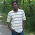## Objective Questions From Basic Electrical Engineering:

 In ac circuit, the ratio of kW/kVA is
A. Power factor
B. Form factor
C. Load factor
D. Diversity factor

 Suppose that two ac waves have the same frequency but differ in phase by exactly 1/20 of a cycle. What is the phase difference between these two waves?
A.  18°
B.  20°
C.  36°
D.  5.73°

 The unit of inductance is
A. Mho
B. Hendry
C. Ohm
D. Farad

 What is the angular frequency of the ac signal having a frequency of 1770Hz
A.  1770 rad/s
B.  11,120 rad/s
C.  282 rad/s
D.  Data insufficient

 Thevenin's equivalent circuit consists of
A. Series combination of Rth, Eth, Rl
B. Series combination of Rth, Eth
C. Parallel combination of Rth, Eth, Rl
D. Parallel combination of Rth, Eth

 The frequency of DC supply is
A. 16 2/3 Hz
B. 60 Hz
C. 50 Hz
D. 0 Hz

 Load factor is defined as the ratio of
A. Max demand/ Average demand
B. Average demand/ Connected load
C. Average demand/ Max. demand
D. Connected load/ Max demand

 Q factor of an inductive coil is given by
A.  2π fL/R
B.  2π fr/R
C.  R/Z
D. lr/l

 The rms value of sinusoidal 100V peak to peak is
A. 100 V
B. 50/√2 V
C. 50 V
D. 100/√2 V

 Which of the following bulbs will have the least resistance?
A. 220V, 60W
B. 220V, 100W
C. 115V, 60W
D. 115V, 100W

Solution Hint:
 One cycle = 360°.
1/20 of cycle is 360/20 = 18°

 Angular frequency ω = 2πf (rad/s)

 Some of the important terms in power system:
Demand factor = maximum demand / Connected load
Diversity factor = Sum of individual max. demands / Max. demand on power station
The ratio of average load to the maximum demand during a given period is known as load factor.
Load Factor = Average Load / maximum demand

 Quality factor is the ratio of reactive power to average power (Q/P)

Qs = [I2XL]/[I2R]
Qs = XL/R
Qs = ωL/R
Q=2π fL/R

 Vrms = Vm/√2

Thanks for reading....
6:00 PM

#### 6 comments:

1.good question thanks *

2.can any one xpln Q.2 solution???

3.I think the answer of qn no 7 is not right. The corre ct answer is c

4.The answer of qn no 8 is also not right i think

5.hi, we regret that for some of the typo errors and they are rectified....solution hint added now for this post and others too..

6.Q9 is wrong they given peak to peak voltage as 100. so peak voltage is 100/2=50 then rms=50/sqrt2